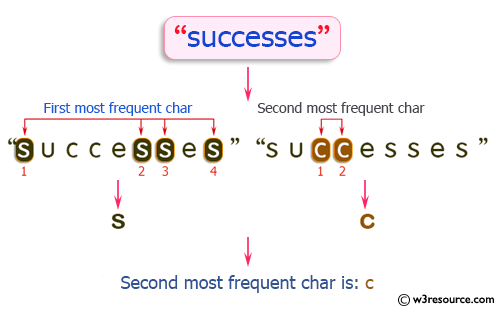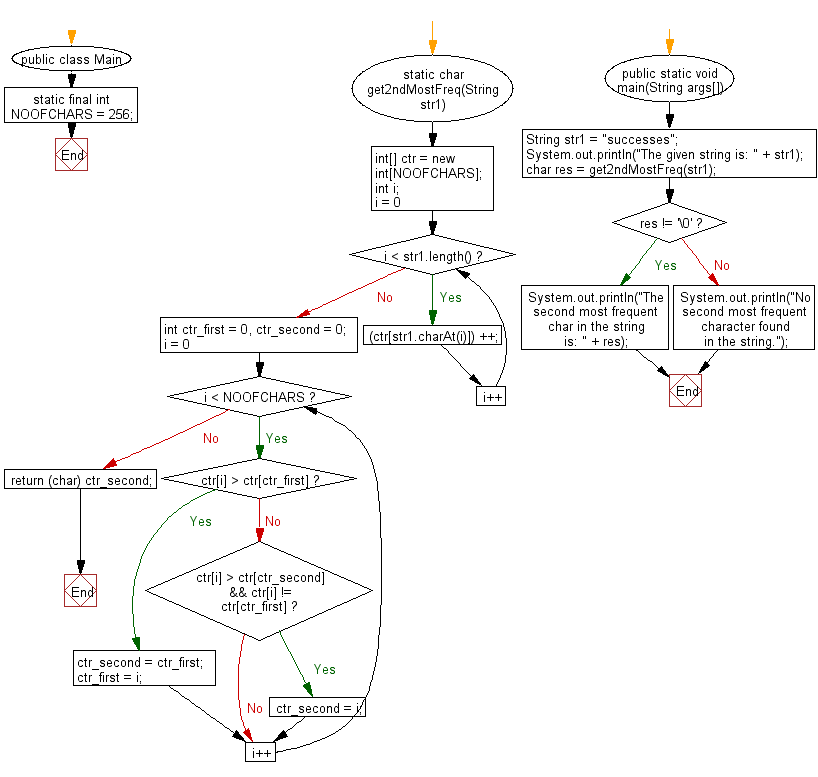﻿ Java exercises: Find the second most frequent character in a given string - w3resource# Java String Exercises: Find the second most frequent character in a given string

## Java String: Exercise-34 with Solution

Write a Java program to find the second most frequent character in a given string.

Pictorial Presentation:Sample Solution:

Java Code:

``````import java.util.*;
public class Main {
static final int NOOFCHARS = 256;
static char get2ndMostFreq(String str1) {

int[] ctr = new int[NOOFCHARS];
int i;
for (i = 0; i < str1.length(); i++)
(ctr[str1.charAt(i)]) ++;

int ctr_first = 0, ctr_second = 0;
for (i = 0; i < NOOFCHARS; i++) {
if (ctr[i] > ctr[ctr_first]) {
ctr_second = ctr_first;
ctr_first = i;
} else if (ctr[i] > ctr[ctr_second] && ctr[i] != ctr[ctr_first])
ctr_second = i;
}
return (char) ctr_second;
}
public static void main(String args[]) {
String str1 = "successes";
System.out.println("The given string is: " + str1);
char res = get2ndMostFreq(str1);
if (res != '\0')
System.out.println("The second most frequent char in the string is: " + res);
else
System.out.println("No second most frequent character found in the string.");
}
}
```
```

Sample Output:

```The given string is: successes
The second most frequent char in the string is: c
```

Flowchart:Java Code Editor:

Improve this sample solution and post your code through Disqus

What is the difficulty level of this exercise?

﻿

## Java: Tips of the Day

Directory Content:

Java allows you to get the names of all subdirectories and files in a folder as an array, which can then be sequentially viewed.

```import java.io.*;

public class ListContents {
public static void main(String[] args) {
File file = new File("//home//user//Documents/");
String[] files = file.list();

System.out.println("Listing contents of " + file.getPath());
for(int i=0 ; i < files.length ; i++)
{
System.out.println(files[i]);
}
}
}
```